Courses
Courses for Kids
Free study material
Free LIVE classes
More

# InterpretingLIVE
Join Vedantu’s FREE Mastercalss

## Introduction

Do you know what interpreting is? Understanding, organising, and analysing the provided data to make sense of it and reach a meaningful conclusion is the data interpretation process. The significance of data interpretation is evident. Data interpretation is arbitrary and differs from company to company. The fundamental idea behind data interpretation is to review the gathered data using analytical techniques and reach suitable conclusions.

## Methods to Interpret Data:

There are two methods to analyse data:

• ### Qualitative Method:

Analysing categories or qualitative data is done using this technique. Texts were used to represent the data in the qualitative data interpretation rather than numbers or patterns. The two types of qualitative data are nominal and ordinal data. Interpreting ordinal data is substantially simpler than interpreting nominal data.

• ### Quantitative Method:

Analysing numerical or quantitative data is done using this method. When interpreting quantitative data, numbers rather than texts represent the data. Discrete and continuous data are the two main types of quantitative data interpretation. Statistical techniques and methods like mean, median, and standard deviation are needed to interpret the data using the quantitative method.

## Types of Interpretation:

Interpretation can be of four types:

• ### Bar Graphs:

We can interpret the relationship between the variables in the shape of rectangular bars by using bar graphs. We might draw these rectangular bars either horizontally or vertically. Bars indicate the many categories of data, and each bar's length corresponds to its value. Grouped, segmented, stacked, and bar graphs are examples.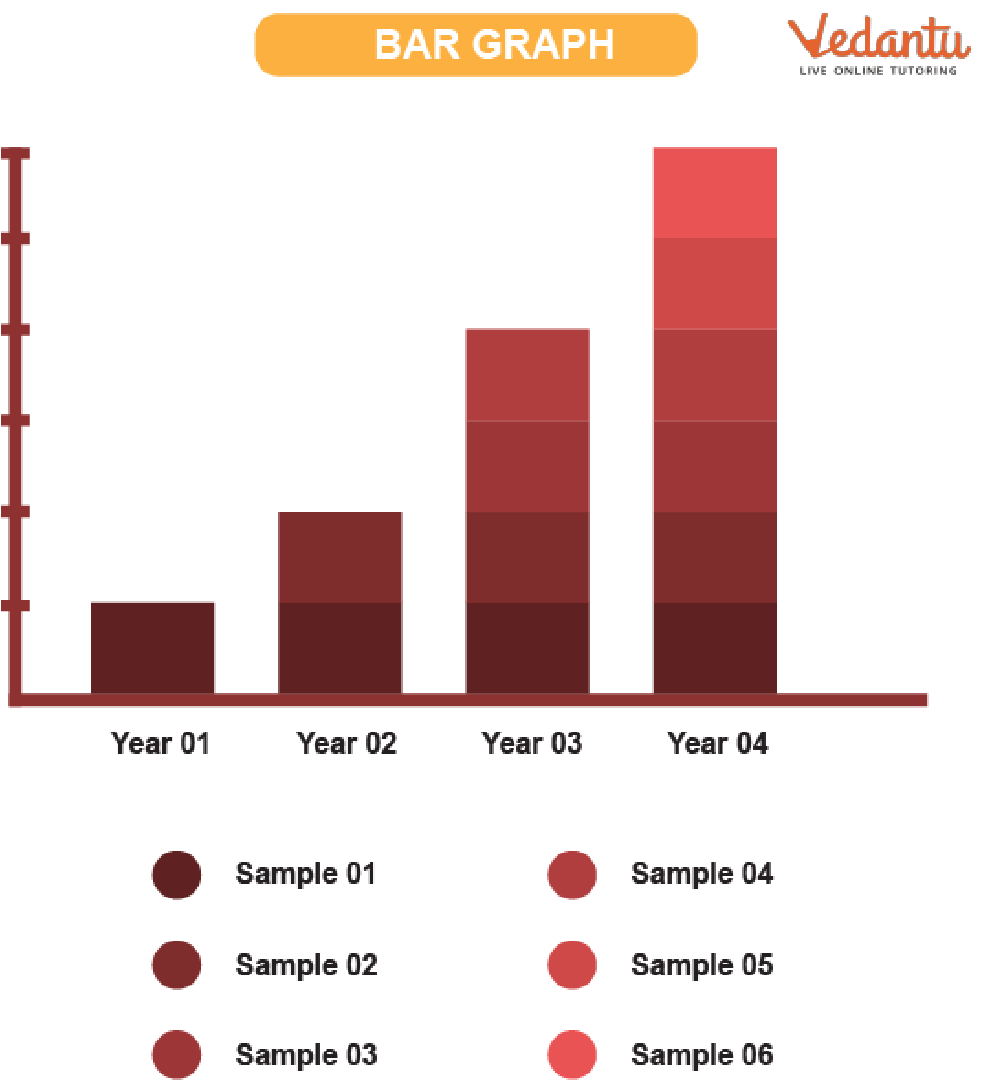Bar Graph

• ### Pie Chart:

A pie chart is a circular diagram showing a variable's proportion. Pie charts show numerical data as percentages or proportions. Simple pie charts, doughnut pie charts, and 3D pie charts are a few varieties of pie charts.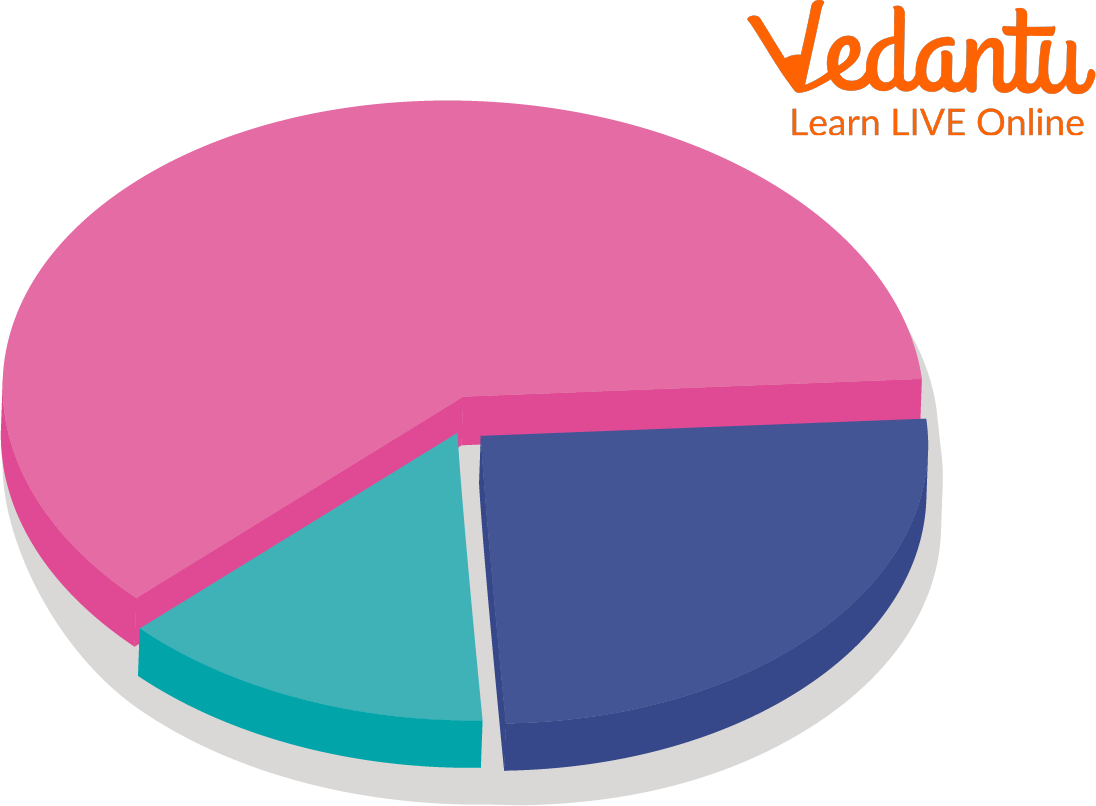Pie Chart

• ### Tables:

Tables are used to display statistical data. Data is organised using rows and columns. Simple tables and complex tables are two different types of tables.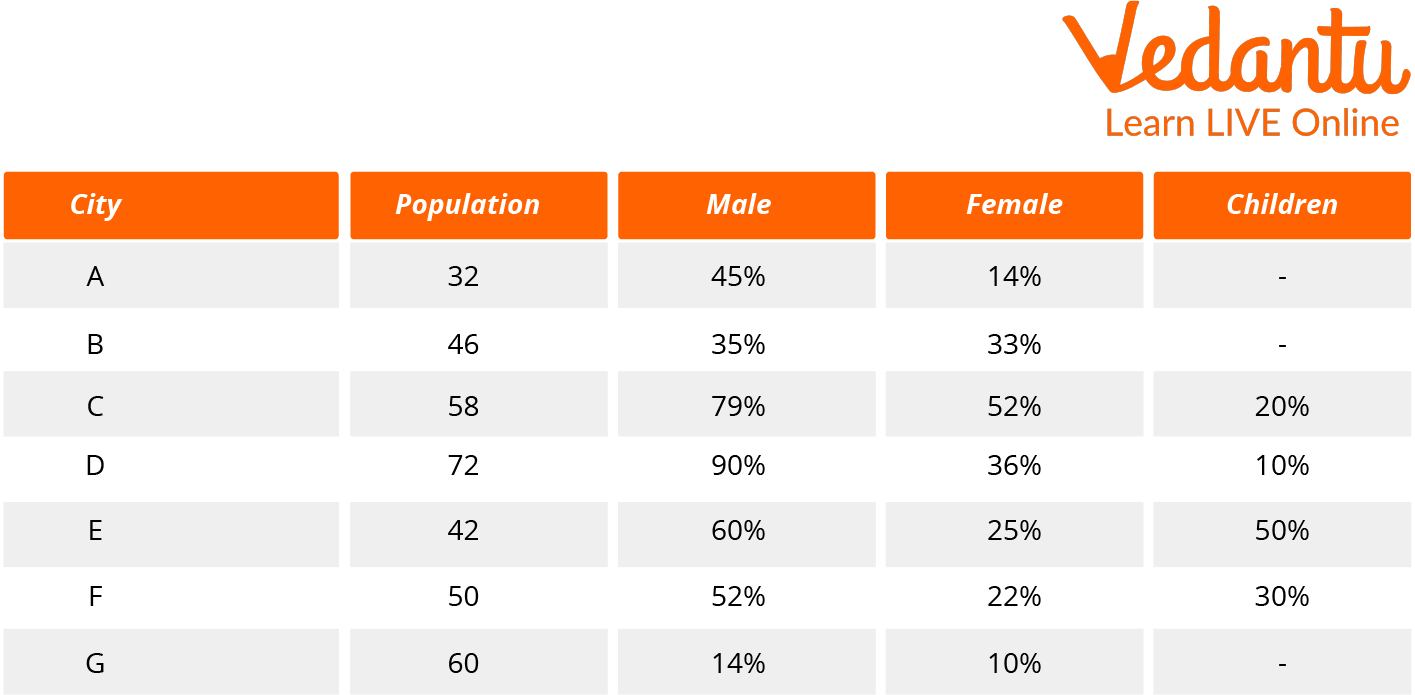Table

• ### Line Graph:

The line graphs comprise the charts or graphs that display data as a series of points. Line charts are excellent for displaying continuous data or a sequence of values. Simple line graphs, stacked line graphs, and other line graphs are among them.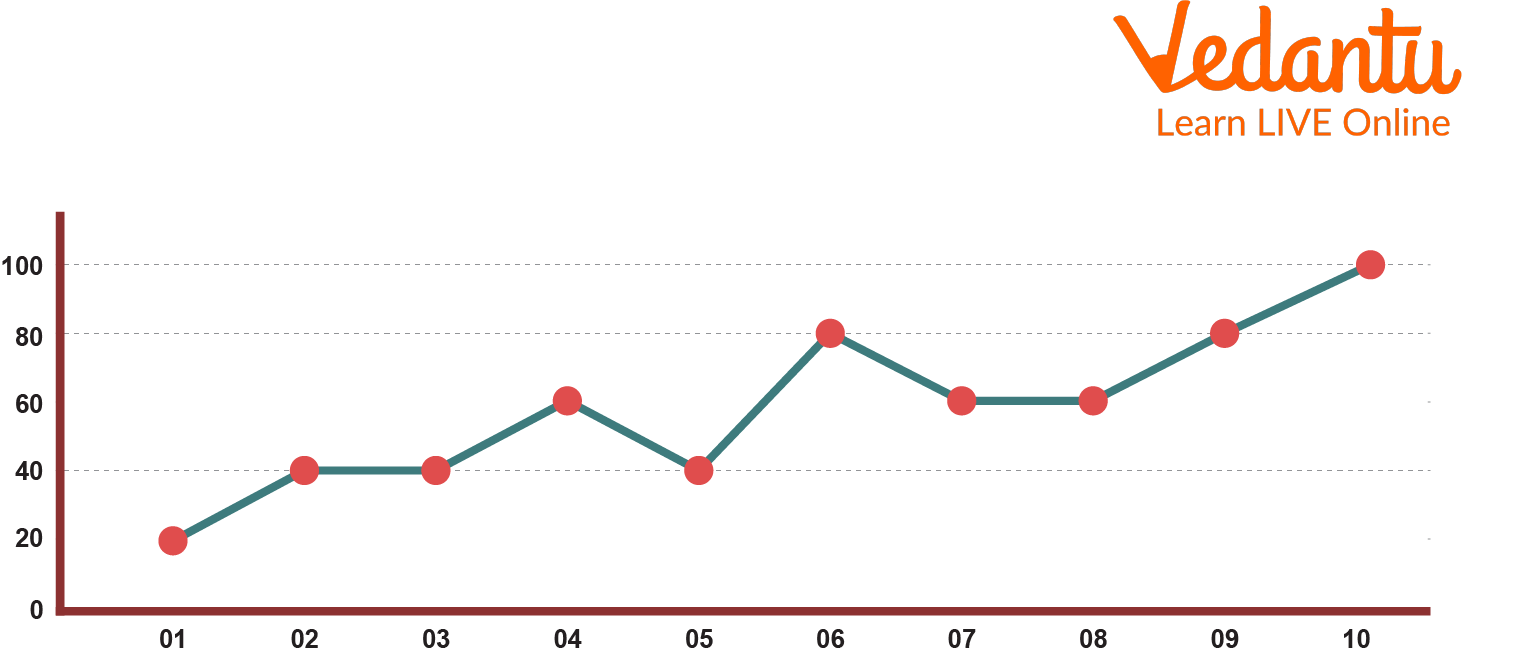Line Graph

## Examples of Interpreting Graphs:

Let us look at some examples of Interpreting Graphs Examples or

Interpreting Graphs, charts, tables, and Diagrams:

### Example :

The cloud symbol represents 25cm of rainfall.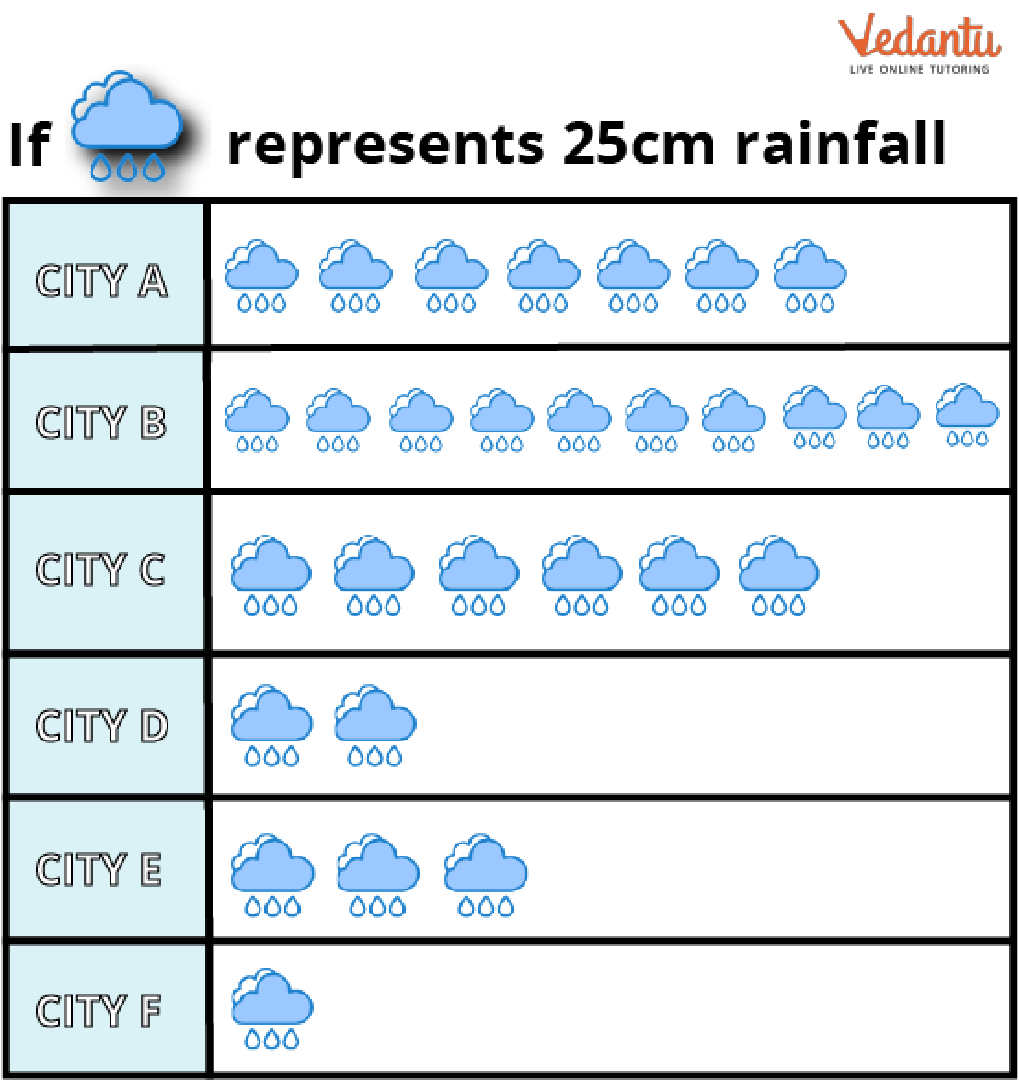1. Give the amount of rainfall in centimetres for the following cities: A, B, C, D, E, and F.

2. Compare the cities B with E's rainfall amounts.

### Solution:

a) From the question, we know 1 cloud symbol represents 25cm of rainfall.

In city A, there are 7 cloud symbols in the pictures.

Rain received in city A$= 25 \times 7 = 175cm$

In city B, there are 10 cloud symbols in the pictures.

Rain received in city B$= 25 \times 10 = 250cm$

In city C, there are 6 cloud symbols in the pictures.

Rain received in city C$= 25 \times 6 = 150cm$

In city D, we can see there are 2 cloud symbols in the pictures.

Rain received in city D$= 25 \times 2 = 50cm$

In city E, there are 3 cloud symbols in the pictures.

Rain received in city E$= 25 \times 3 = 75cm$

In city F, we can see 1 cloud symbol in the pictures.

Rain received in city F$= 25 \times 1 = 25cm$

b) We can see from the last part the rainfall in city B is 250cm, and in city E, it is 75cm. Therefore, we can say that city B recorded more rain than city E.

## Conclusion:

Data interpretation is examining data using analytical techniques that will give the data some meaning and lead to a helpful conclusion. The fundamental idea behind data interpretation is that it focuses significantly on statistical knowledge, particularly data analysis. Every field can benefit from data interpretation. The gathering of data has advantages for a variety of organisations and people.

Last updated date: 26th Sep 2023
Total views: 78.3k
Views today: 0.78k

## FAQs on Interpreting

1. How do you teach a young child about data handling?

The most excellent method for working with young in data handling is to help them gather their facts and then demonstrate how to accurately record them, whether in a list, table, or tally chart. Teachers then explain the process of turning this data into a pictogram or block diagram.

2. Why is data relevant to children?

Improved problem-solving abilities are only one of the many advantages of teaching data science to children. Kids can think creatively and carefully using data science, which is essential for problem-solving.

3. Why is data so necessary in modern life?

We can assist individuals and support them to live better lives by using data: Organizations should use data for various reasons, but improving quality should be their primary focus. An efficient data system can help your organisation improve the quality of people's lives by enabling measurement and action.